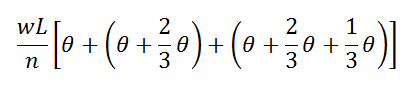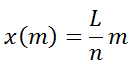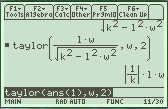# Beam Bending 3

We earlier assumed all the hinge angles were equal, simply to get an answer as easily as possible.  Now we can question that.

It might make sense that, assuming energy is conserved with gravitational equals spring potential energy, that we might add another condition, that potential energy is at an extreme point.

The idea that physics fits into extremes is an old general principle, example, the principle of least distance (law of reflection), principle of least time (refraction), principle of maximum entropy, principle of least action (almost everything).

However, we can justify it a little more in this case.  If GPE and SPE are balanced, but the spring could lower its potential energy by changing the exact way it is bent, would it?  Of course.

The general conservation of energy equation isThen, with that constraint, we want to maximize the right side.  We can do that with the technique of Lagrangian Multipliers.

We define the auxiliary functionThen the gradient of F must be zero.

I usually think of F as a function of the thetas only, but if you consider it a function of lambda as well, the lambda component of its gradient is simply the original constraint (which I otherwise think of separately).  Whatever.

Note that the assumption of maximum SPE always gives us enough equations to solve the system.

Anyway, the gradient of F being zero introduces the equationswhere the index t can be any integer between 1 and n.

So now we have the equations — the only problem is how hard they are to solve!  So we still have to make a simplifying assumption.  We assume all the angles are very small, so sinz = z and cosz = 1, even in cases where the argument is the sum of multiple angles.

This simplifies the previous equation toThe savings of this simplification are phenomenal: The thetas aren’t even coupled anymore!  (ie., only one theta occurs in each equation).

This lets us immediately solve for any theta in terms of the first one:Note how different this is from the previous guess: Far from being equal, the angles decrease as you approach the weighted end.

Now we can solve for theta1 by plugging back into equation .  It first seems we might have to do a sum of a series of squares and head back to Bernoulli Numbers, but when we simplify the sin term on the right with sinz = z, the same sum occurs on both sides.

To see why, consider, say, n = 3.The theta term occurs n times, but put it over a common denominator of n and it’s n^2.  The 2/3 term has a factor of (n-1) and occurs (n-1) times.  The 1/3 term has a factor of (n-2) and occurs (n-2) times.  It’s a sum of squares.  The only thing is, on the right side, the squares are over a denominator of n, while on the left they are over a denominator of n^2.

So, theta1 isThis is twice the theta value obtained in the previous post; however, since each subsequent theta decreases, it turns out that the sum of all thetas in both cases is the same (wL/k)

We’ve almost solved the problem; we just might want the explicit curve of x and y.

x and y are given byWhen we make the small angle approximation, x simplifies trivially (if we had to guess in one step what it would be, it would be this).As for y, it’s more complicated; we almost do need a sum of squares.But screw it, the TI89 can handle that sum.(if you had to do it yourself, you could do it through, say, interpolation, since you know the answer must be a polynomial)

Hell, let’s just finish this up (substitute for m and take the limit) on the TI89:So the final curve isIn fact, this is exactly the canonical result you would get from Euler-Bernoulli Beam theory, so long as you identify k as(Is that reasonable?  Let’s leave that for another time)

We can also check the consistency of one of our assumptions, that these beams act like hinges that obey Hooke’s Law.

The slope at the “wall” end is 0, the slope at the free end is wL/k.  This is the angle the “hinge” is bent into, assuming it’s small (tanz~z)

Hooke’s Law was F = w = k’ theta = (k/L) theta = (k/L) (wL/k) = w.

So it checks out.

The answer is the same for the other assumption in the limit that w goes to zero, but otherwise not.With that said, we can also compare and contrast curves drawn with the earlier assumption and the correct assumption.In this case, w/k= 0.1

It turns out that as w/k tends to zero, the correct end deflection is 4/3 times greater than that given with the earlier guess.

With increasing w/k, the ratio decreases.The above is with w/k=0.8.

The earlier (wild ass guess) is not completely terrible, in terms of getting qualitative answers.  Basically, what I am actually going to be doing is just trying to predict that the expected neck bending is acceptably negligible, which is almost inherently a qualitative criteria.

So even though we did this problem completely differently than Wikipedia would seem to suggest, we ultimately got the same answer.  (Not only did we not use a differential equation, we also did not need to reason out the boundary conditions — they more or less popped out automatically)  I don’t know if there is anywhere else on the internet that approaches this problem in this manner.

Ultimately, I think looking at energy rather than force might make this approach a more natural fit to the problem of solving for the bend of a guitar neck anyway.

If we look at force, the lines of force (tension) on the strings are parallel to the neck, so they should only compress the neck, not bend it.

However, this is like saying that gravity can never topple a pencil balanced on its point, because the gravitational force is exactly balanced by the normal force supporting it. But look at energy and you can see it’s an unstable equilibrium.

(This reminds me, back when I was in school, I would sometimes try to numerically model (to get extra insight into) problems like a ladder resisting against a wall, then sliding down it, and solve for when the ladder leaves the wall. I think I did do this with direct time integration of Newton’s 2nd Law; since a computer interprets it literally, you have to start with the ladder slightly tilted or it will truly never fall)

And for the guitar neck, since the strings are in tension, they can lose energy if they can shorten. If bending of the guitar neck allows the strings to shorten, it will happen (until the energy lost by shortening the strings equals the energy gained by bending the neck). You don’t have to worry so much about the kinectics of how the bending gets started to begin with.

## 2 thoughts on “Beam Bending 3”

1.admin on said:

I mentioned “maximizing” the SPE; I really just meant making it an extreme value.

2.admin on said:

“If bending of the guitar neck allows the strings to shorten, it will happen ”

note here however that the small angle approx means x doesn’t change while y increases; this will only increase the length of the string, so some higher order terms will be necessary. (the “bad” approx. did not use small angle and does allow for decreasing x)